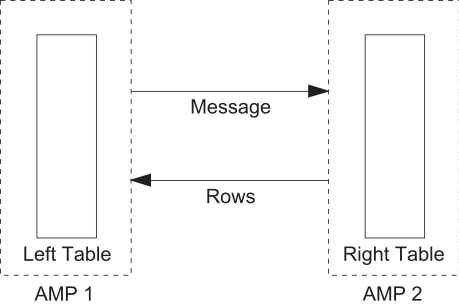# 16.10 - Remote Nested Join - Teradata Database

## Teradata Database SQL Request and Transaction Processing

prodname
vrm_release
16.10
created_date
June 2017
category
Programming Reference
User Guide
featnum
B035-1142-161K

## Definition of the Remote Nested Join

Remote nested join is used for the case in which a WHERE condition specifies a constant value for a unique index of one table, and the conditions might also match some column of that single row to the primary or secondary index of a second table.

The expression remote nested join implies that a message is to be sent to another AMP to get the rows from the right table.

A remote nested join does not always use all AMPs. For this reason, it is the most efficient join in terms of system resources and is almost always the best choice for OLTP applications.

Remote nested joins normally avoid the duplication or redistribution of large amounts of data and minimize the number of AMPs involved in join processing.

The following SELECT request is an example of a remote nested join in that no join condition exists between the two tables:

```   SELECT *
FROM table_1, table_2
WHERE table_1.USI_1
AND   table_2.USI_2 = 1;```

When there is no such join condition, then the index of the second (right) table must be defined with a constant as illustrated by Examples 1, 2, and 3 if a remote nested join is to be used.

## How a Remote Nested Join Is Processed

The process applied by the remote nested join is as follows:
1. Read the single left row.
2. Evaluate the index value for the right table.
3. Read the right table rows using the index value.
4. Produce the join result.

## Remote Nested Join Process

Remote nested join is used for the condition where one table contains the key to the table with which it is to be joined. The key can be of any of the following database objects:
• Unique primary index (UPI)
• Nonunique primary index (NUPI)
• Unique secondary index (USI)
• Nonunique secondary index (NUSI)
• Unindexed column that is matched to an index
If there is such a join condition, and the conditions of the first table match a column of the primary or secondary index of the second table, then the following process occurs:
1. Retrieve the single qualifying row from the first table.
2. Use the row hash value to locate the AMP having the matching rows in the second table to make the join.

The following graphic illustrates the remote nested join process:## Examples of Remote Nested Join Conditions

A remote nested join can be used when there is no equality condition between the primary indexes of the 2 tables and other conditions. This is illustrated by the following example conditions:

```       (table_1.UPI = constant OR table_1.USI = constant)
AND (table_2.UPI = constant OR table_2.USI = constant)```

In this case, there may or may not be a suitable join condition.

The following 2 examples illustrate cases where there is a suitable join condition:

```        (table_1.UPI = constant OR table_1.USI = constant)
AND ((table_2.NUPI = table_1.field)
OR (table_2.USI = table_1.field))

(table_1.NUPI = constant)
AND (table_2.UPI = table_1.field)
AND (few_rows_returned_from_the_table_1.NUPI = constant)```